# 3.2 powerpoint

28 Oct 2012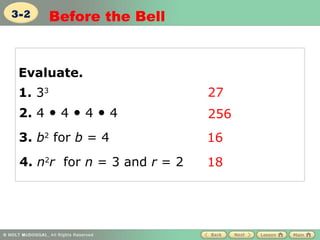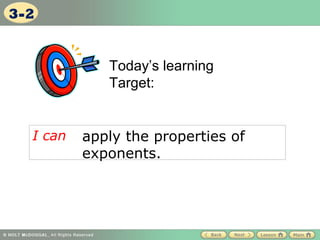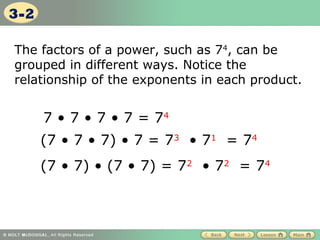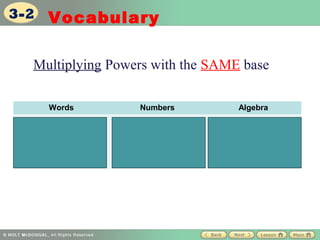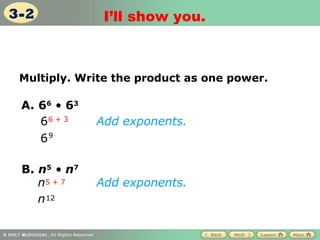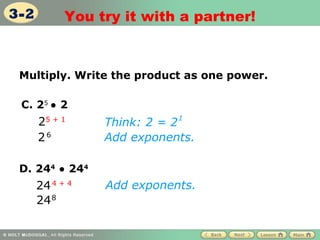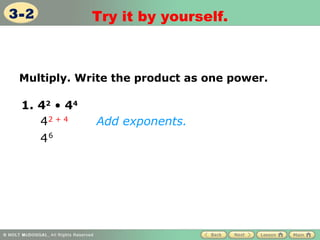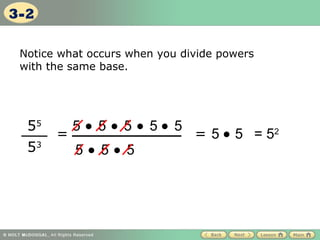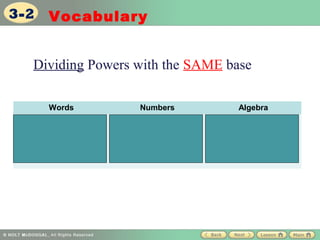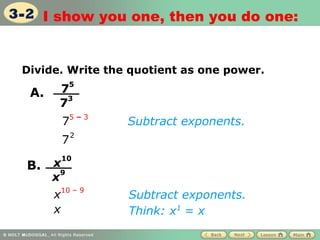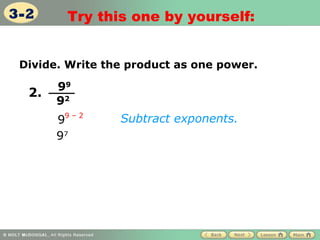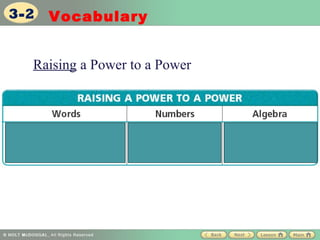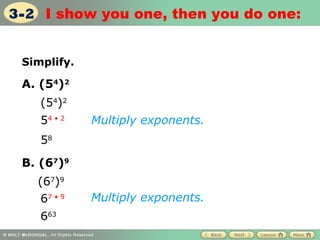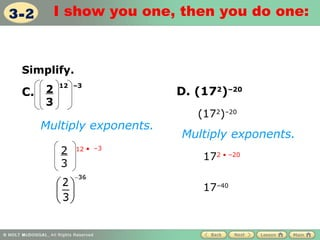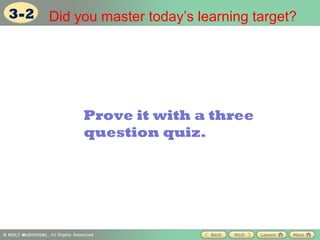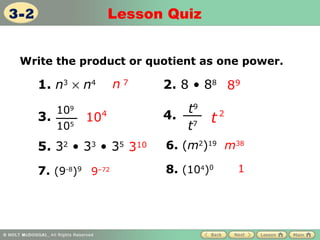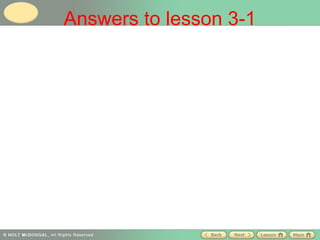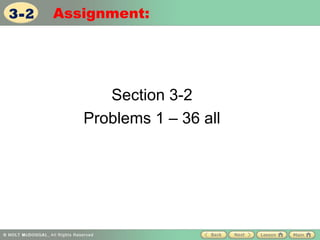1 sur 18

### 3.2 powerpoint

• 1. 3-2 Before the Bell Evaluate. 1. 33 27 2. 4 • 4 • 4 • 4 256 3. b2 for b = 4 16 4. n2r for n = 3 and r = 2 18
• 2. 3-2 Today’s learning Target: I can apply the properties of exponents.
• 3. 3-2 The factors of a power, such as 74, can be grouped in different ways. Notice the relationship of the exponents in each product. 7 • 7 • 7 • 7 = 74 (7 • 7 • 7) • 7 = 73 • 71 = 74 (7 • 7) • (7 • 7) = 72 • 72 = 74
• 4. 3-2 Vocabulary Multiplying Powers with the SAME base Words Numbers Algebra Keep the base 35 3 8 bm b n and add the 35+8 = bm+n exponents 313
• 5. 3-2 I’ll show you. Multiply. Write the product as one power. A. 66 • 63 66 + 3 Add exponents. 69 B. n5 • n7 n5 + 7 Add exponents. n 12
• 6. 3-2 You try it with a partner! Multiply. Write the product as one power. C. 25 • 2 25 + 1 Think: 2 = 2 1 26 Add exponents. D. 244 • 244 24 4 + 4 Add exponents. 24 8
• 7. 3-2 Try it by yourself. Multiply. Write the product as one power. 1. 42 • 44 42 + 4 Add exponents. 46
• 8. 3-2 Notice what occurs when you divide powers with the same base. 55 5• 5• 5• 5• 5 = = 5 • 5 = 52 53 5• 5• 5
• 9. 3-2 Vocabulary Dividing Powers with the SAME base Words Numbers Algebra Keep the base 38 = 38-2 = 36 bm = bm-n and subtract the 32 bn exponents
• 10. 3-2 I show you one, then you do one: Divide. Write the quotient as one power. 5 A. 7 3 7 75 – 3 Subtract exponents. 2 7 10 B. x9 x x10 – 9 Subtract exponents. x Think: x1 = x
• 11. 3-2 Try this one by yourself: Divide. Write the product as one power. 99 2. 92 99 – 2 Subtract exponents. 97
• 12. 3-2 Vocabulary Raising a Power to a Power
• 13. 3-2 I show you one, then you do one: Simplify. A. (54)2 (54)2 54 • 2 Multiply exponents. 58 B. (67)9 (67)9 67 • 9 Multiply exponents. 663
• 14. 3-2 I show you one, then you do one: Simplify. 12 –3 C. 2 D. (172)–20 3 (172)–20 Multiply exponents. Multiply exponents. 2 12 • –3 172 • –20 3 17–40
• 15. 3-2 Did you master today’s learning target? Prove it with a three question quiz.
• 16. 3-2 Lesson Quiz Write the product or quotient as one power. 1. n3 × n4 n7 2. 8 • 88 89 109 t9 3. 104 4. t2 105 t7 5. 32 • 33 • 35 310 6. (m2)19 m38 7. (9-8)9 9–72 8. (104)0 1
• 17. Answers to lesson 3-1
• 18. 3-2 Assignment: Section 3-2 Problems 1 – 36 all